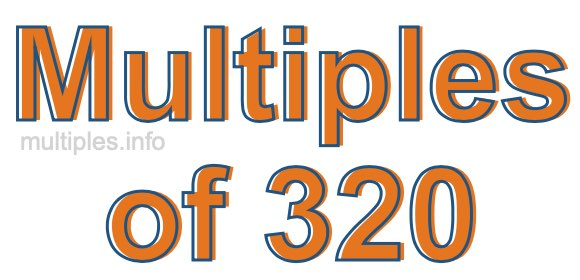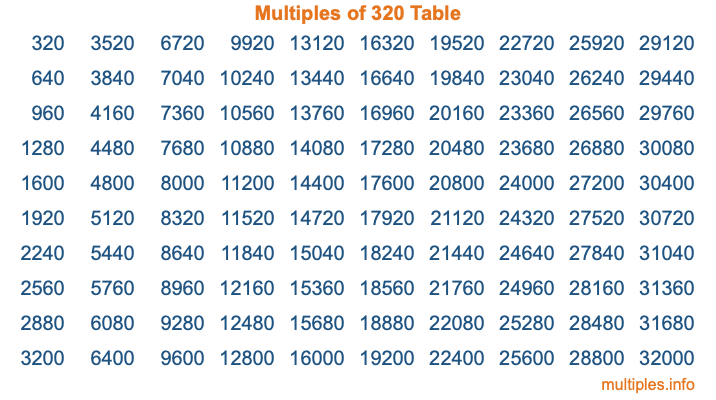Multiples of 320Welcome to the Multiples of 320 page. Here we will first teach you everything you will ever need to know about the multiples of 320, and then give you a study guide summary of everything we taught you to make sure you remember it all. Use this page to look up facts and learn information about the multiples of 320. This page will make you a multiples of three hundred twenty expert!

Definition of Multiples of 320
Multiples of 320 are all the numbers that when divided by 320 equal an integer. Each of the multiples of 320 are called a multiple. A multiple of 320 is created by multiplying 320 by an integer.

Therefore, to create a list of multiples of 320, you start with 1 multiplied by 320, then 2 multiplied by 320, then 3 multiplied by 320, and so on for as long as you want. Thus, the list of the first five multiples of 320 is 320, 640, 960, 1280, and 1600. To see a larger list of multiples of 320, see the printable image of Multiples of 320 further down on this page. We also have a category where you can choose any nth multiple of 320.

Multiples of 320 Checker
The Multiples of 320 Checker below checks to see if any number of your choice is a multiple of 320. In other words, it checks to see if there is any number (integer) that when multiplied by 320 will equal your number. To do that, we divide your number by 320. If the the quotient is an integer, then your number is a multiple of 320.

Is  a multiple of 320?

Least Common Multiple of 320 and ...
A Least Common Multiple (LCM) is the lowest multiple that two or more numbers have in common. This is also called the smallest common multiple or lowest common multiple and is useful to know when you are adding our subtracting fractions. Enter one or more numbers below (320 is already entered) to find the LCM.

Check out our LCM Calculator if you need more details about the Least Common Multiple or if you need the LCM for different numbers for adding and subtraction fractions.

nth Multiple of 320
As we stated above, 320 is the first multiple of 320, 640 is the second multiple of 320, 960 is the third multiple of 320, and so on. Enter a number below to find the nth multiple of 320.

th multiple of 320

Multiples of 320 vs Factors of 320
320 is a multiple of 320 and a factor of 320, but that is where the similarities end. All postive multiples of 320 are 320 or greater than 320. All positive factors of 320 are 320 or less than 320.

Below is the beginning list of multiples of 320 and the factors of 320 so you can compare:

Multiples of 320: 320, 640, 960, 1280, 1600, etc.

Factors of 320: 1, 2, 4, 5, 8, 10, 16, 20, 32, 40, 64, 80, 160, 320

As you can see, the multiples of 320 are all the numbers that you can divide by 320 to get a whole number. The factors of 320, on the other hand, are all the whole numbers that you can multiply by another whole number to get 320.

It's also interesting to note that if a number (x) is a factor of 320, then 320 will also be a multiple of that number (x).

Multiples of 320 vs Divisors of 320
The divisors of 320 are all the integers that 320 can be divided by evenly. Below is a list of the divisors of 320.

Divisors of 320: 1, 2, 4, 5, 8, 10, 16, 20, 32, 40, 64, 80, 160, 320

The interesting thing to note here is that if you take any multiple of 320 and divide it by a divisor of 320, you will see that the quotient is an integer.

Multiples of 320 Table
Below is an image of the first 100 multiples of 320 in a table. The table is in chronological order, column by column. The first column has the first ten multiples of 320, the second column has the next ten multiples of 320, and so on.The Multiples of 320 Table is also referred to as the 320 Times Table or Times Table of 320. You are welcome to print out our table for your studies.

Negative Multiples of 320
Although not often discussed or needed in math, it is worth mentioning that you can make a list of negative multiples of 320 by multiplying 320 by -1, then by -2, then by -3, and so on, to get the following list of negative multiples of 320:

-320, -640, -960, -1280, -1600, etc.

Multiples of 320 Summary
Below is a summary of important Multiples of 320 facts that we have discussed on this page. To retain the knowledge on this page, we recommend that you read through the summary and explain to yourself or a study partner why they hold true.

There are an infinite number of multiples of 320.

A multiple of 320 divided by 320 will equal a whole number.

320 divided by a factor of 320 equals a divisor of 320.

The nth multiple of 320 is n times 320.

The largest factor of 320 is equal to the first positive multiple of 320.

320 is a multiple of every factor of 320.

320 is a multiple of 320.

A multiple of 320 divided by a divisor of 320 equals an integer.

320 divided by a divisor of 320 equals a factor of 320.

Any integer times 320 will equal a multiple of 320.

Multiples of a Number
Here you can get the multiples of another number, all with the same attention to detail as we did for multiples of 320 on this page.

Multiples of
Multiples of 321
Did you find our page about multiples of three hundred twenty educational? Do you want more knowledge? Check out the multiples of the next number on our list!